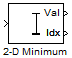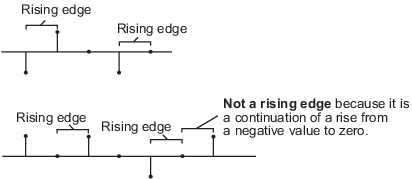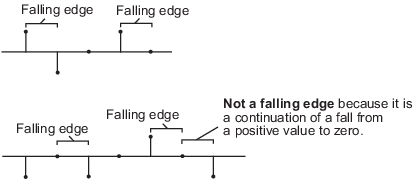# 2-D Minimum

Find minimum values in input or sequence of inputs

• Library:
• Computer Vision Toolbox / Statistics

•## Description

The 2-D Minimum block identifies the value, and optionally the position, of the smallest element in the input. The input can be a vector, a matrix, or an N-D array. The block identifies the minimum value either along a specified dimension of the input or across the entire input. It also tracks the minimum values in a sequence of inputs over a period of time when the Mode parameter is set to `Running`.

## Ports

### Input

expand all

Input array, specified as a vector, matrix, or N-D array.

#### Dependencies

The port is named only when you either select Enable ROI processing parameter or set the Mode parameter to `Running`.

Data Types: `single` | `double` | `int8` | `int16` | `int32` | `uint8` | `uint16` | `uint32` | `fixed point`
Complex Number Support: Yes

Reset the running minimum, specified as a scalar. This port specifies the event that causes the block to reset the running minimum. The sample time of the Rst input must be a positive integer and a multiple of the block input sample time.

#### Dependencies

To enable this port, set the Mode parameter to `Running` and set the Reset port parameter to `Rising edge`, ```Falling edge```, `Either edge`, or `Non-zero sample`.

Data Types: `single` | `double` | `int8` | `int16` | `int32` | `uint8` | `uint16` | `uint32` | `Boolean`

Region of interest (ROI), specified as a four-element vector, m-by-4 matrix, or M-by-N matrix. This port accepts different input values depending on the setting of the ROI type parameter.

Note

• You can use the ROI port only if the input to the In port is a 2-D image.

• You cannot use the ROI port if the Mode parameter is set to `Running`.

#### Dependencies

To enable this port, set the Find the minimum value over parameter to `Entire input` and select the Enable ROI processing parameter.

Data Types: `single` | `double` | `int8` | `int16` | `int32` | `uint8` | `uint16` | `uint32` | `Boolean` | `fixed point`

Label matrix, specified as a matrix of nonnegative integers. The label matrix represents the objects in a 2-D image. The pixels labeled `0` are the background. The pixels labeled `1` make up the first object, the pixels labeled `2` make up the second object, and so on. The size of the label matrix must be same as the size of the 2-D input image.

#### Dependencies

To enable this port, select the Enable ROI processing parameter and set the ROI type parameter to `Label matrix`.

Note

You cannot enable the Label port if the Mode parameter is set to `Running`.

Data Types: `uint8` | `uint16` | `uint32`

Label values of ROI, specified as an M-element vector. This represents the object names for the corresponding numbers in the label matrix. M must be less than or equal to the number of objects in the label matrix.

#### Dependencies

To enable this port, select the Enable ROI processing parameter and set the ROI type parameter to `Label matrix`.

Note

You cannot enable the `Label Numbers` port if the Mode parameter is set to Running.

Data Types: `uint8` | `uint16` | `uint32`

### Output

expand all

Minimum values of the input, returned as a scalar, vector, matrix, or N-D array. The size of this output depends on the size of the input, and the settings of the Mode and Find the minimum value over parameters.

Note

This port is unnamed if the Mode parameter is set to `Running`. It doesn't appear if the Mode parameter is set to `Index`.

#### Compute Minimum Value of Input Array

Set the Mode parameter to ```Value and Index``` or `Value`. The block computes the minimum value along the specified dimension of the input or across the entire input. The size of the output minimum value depends on the size of the input and the setting of the Find the Minimum value over parameter.

• Scalar — The input is of any size, and the Find the minimum value over parameter is set to ```Entire input```.

• Vector — The input is a matrix, and the Find the minimum value over parameter is set to ```Each row```, `Each column`, or `Specified dimension`. If ```Specified dimension``` is selected, the value of the Dimension parameter must be either `1` or `2`.

• (N–1)-D array — The input is an N-D array, the Find the minimum value over parameter is set to `Specified dimension`, and the value of the Dimension parameter is N.

• N-D array with one singleton dimension — The input is an N-D array, and the Find the minimum value over parameter is set to `Each row`, `Each column`, or ```Specified dimension```. If `Specified dimension` is selected, the value of the Dimension parameter must be an integer less than N.

Example: For a 3-D input array of size M-by-N-by-P, the dimension of the returned output is:

• 1-by-N-by-P if you set the Find the minimum value over parameter to `Entire row`.

• M-by-1-by-P if you set the Find the minimum value over parameter to `Entire column`.

• M-by-N if you set the Find the minimum value over parameter to `Specified dimension` and the Dimension parameter to `3`.

#### Compute Minimum Value of Sequence of Inputs

Set the Mode parameter to `Running`. The block finds the minimum value of all the inputs in the given sequence and compiles them into a single array. The output is of the same size as the input.

Data Types: `single` | `double` | `int8` | `int16` | `int32` | `uint8` | `uint16` | `uint32` | `fixed point`

Indices of the minimum values in the input array, returned as a scalar, vector, matrix, or N-D array.

To enable this port, set the Mode parameter to `Value and Index` or `Index`.The size of the output depends on the size of the input and the setting of the Find the minimum value over parameter.

• Scalar — The input is an N-element vector, and the Find the minimum value over parameter is set to `Entire input`.

• Vector — The input is a matrix, and the Find the minimum value over parameter is set to ```Entire input```, `Each row`, ```Each column```, or `Specified dimension`. If `Specified dimension` is selected, the value of the Dimension parameter must be either `1` or `2`.

• (N–1)-D array — The input is an N-D array, the Find the minimum value over parameter is set to `Specified dimension`, and the value of the Dimension parameter is N.

• N-D array with one singleton dimension — The input is an N-D array, and the Find the minimum value over parameter is set to `Each row`, `Each column`, or ```Specified dimension```.If `Specified dimension` is selected, the value of the Dimension parameter must be an integer less than N.

Example: For a 3-D input array of size M-by-N-by-P, the dimension of the returned output is:

• 1-by-N-by-P if you set the Find the minimum value over parameter to `Entire row`.

• M-by-1-by-P if you set the Find the minimum value over parameter to `Entire column`.

• M-by-N if you set the Find the minimum value over parameter to `Specified dimension` and the Dimension parameter to `3`.

Note

When a minimum value occurs more than once, the computed index corresponds to the first occurrence. For example, if the input vector is ```[3 2 1 2 1]```, then the minimum value is `1` and the one-based index of the minimum value is `3`.

Data Types: `single` | `double` | `int8` | `int16` | `int32` | `int64` | `uint8` | `uint16` | `uint32` | `uint64` | `fixed point`

ROI Validation, returned as a scalar or vector of logical `1`s (`true`) or `0`s (`false`). If the ROI type parameter is set to `Rectangles` or `Lines`, the output signifies whether or not the specified ROIs lie completely or partially within the input image. If the ROI type parameter is set to `Label matrix`, the output signifies whether or not the label numbers specified in the Label Numbers input are present in the input label matrix.

 ROI type Individual statistics for each ROI Single statistics for all ROIs `Rectangles` The port returns an m element vector, wherem is the number of rows in the m-4 matrix in the input to the ROI port. Each element of this vector is a `1` or `0`, indicating that the rectangular ROI is either completely or partially inside the input image (`1`) or completely outside the input image (`0`). The port returns a scalar. The scalar is a `1` or `0`, indicating that any of the rectangles in the ROI input is present completely or partially inside (`1`) or completely outside (`0`) the input image. `Lines` The port returns a scalar. The scalar is a `1` or `0`, indicating that the input given to the ROI port is either completely or partially inside (`1`) the input image or completely outside (`0`) the input image. The port returns a scalar. The scalar is a `1` or `0`, indicating that the input given to the ROI port is either completely or partially inside (`1`) the input image or completely outside (`0`) the input image. `Label matrix` The port returns an M-element vector, where M is the number of elements in the input to the Label Numbers port. Each element of the vector is a `1` or `0`, indicating that the associated label from the Label Numbers input is present in (`1`) or absent from (`0`) the Label Matrix input. The port returns a scalar. The scalar is a `1` or `0`, indicating that any of the labels in the Label Numbers input are present in (`1`) or all are absent from (`0`) the Label Matrix input.

Note

If the ROI is partially outside the image, the block computes the statistical values for only the portion of the ROI that lies within the image bounds.

#### Dependencies

To enable this port, select the Output flag indicating if ROI is within image bounds parameter and set the value of the ROI type parameter to `Rectangle`, or `Lines`, or select the Output flag indicating if input label numbers are valid parameter and set the value of ROI type parameter to```Label matrix```.

## Parameters

expand all

Main

Specify the output mode of the block as one of these options.

• `Value and Index` — Return both the minimum values and their corresponding indices in the given input.

• `Value` — Return only the minimum values.

• `Index` — Return only the indices of the minimum values in the given input.

• `Running` — Tracks the minimum values of all input elements across a sequence of inputs minimum values in a sequence of inputs.

Specify the index for the first element in the input array.

• `One` for one-based numbering. The range of index values for each dimension is 1 to m, where m is the length of that dimension. For example, the index of the first element in a matrix is (`1,1`).

• `Zero` for zero-based numbering. The range of index values for each dimension is 0 to m–1, where m is the length of that dimension. For example, the index of the first element in a matrix is (`0,0`).

Specify the dimension of the input along which the block computes the minimum.

• `Entire input` — Computes minimum over the entire input.

• `Each row` — Computes minimum over each row.

• `Each column` — Computes minimum over each column.

• `Specified dimension`— Computes minimum over the dimension specified in the Dimension parameter.

• If the Dimension parameter is set to `1`, the output is the same as when ```Each column``` is selected.

• If the Dimension parameter is set to `2`, the output is the same as when ```Each row``` is selected.

#### Dependencies

To enable this parameter, set the Mode parameter to `Value and Index`, `Value`, or `Index`.

Specify the dimension of the input array over which the block computes the minimum as a one-based value. The value of this parameter must be greater than zero and less than or equal to the number of dimensions in the input array.

#### Dependencies

To enable this parameter, set the Find the minimum value over parameter to `Specified dimension`.

Specifies what the block detects as a reset event. The block resets the running minimum when a reset event is detected at the Rst port. The reset sample time must be a positive integer and a multiple of the input sample time.

Specify the reset event as one of these options.

• `None` — Disable the Rst port.

• `Rising edge` — Trigger a reset event when the Rst input does one of the following.

• Rises from a negative value to either a positive value or zero

• Rises from zero to a positive value, where the rise is not a continuation of a rise from a negative value to zero• `Falling edge` — Trigger a reset event when the Rst input does one of the following.

• Falls from a positive value to either a negative value or zero

• Falls from zero to a negative value, where the fall is not a continuation of a fall from a positive value to zero• `Either edge` — Trigger a reset event when the Rst input is either a ```Rising edge``` or a `Falling edge`.

• `Non-zero sample` — Trigger a reset event at each sample time, where the Rst input is not zero.

Note

When running simulations in Simulink® multitasking mode, reset signals have a one-sample latency. In this case, when the block detects a reset event, there is a one-sample delay at the Rst port rate before the block applies the reset.

#### Dependencies

To enable this parameter, set the Mode parameter to `Running`.

Select to calculate the minimum within an ROI in the image.

Note

Full ROI processing is available only if you have a Computer Vision Toolbox™ license. If you do not have a Computer Vision Toolbox license, you can still use ROI processing, but the ROI type parameter is limited to `Rectangles`.

#### Dependencies

To enable this parameter, set the Find the minimum value over parameter to `Entire input`.

Specify the ROI format that represents the regions in the image over which to compute the minimum. The type of ROI can be a rectangle, line, label matrix, or a binary mask.

 ROI type Inputs to the ROI port Description `Rectangles` Four-element row vector [x y width height]m-by-4 matrix : `$\left[\begin{array}{cccc}{x}_{1}& {y}_{1}& widt{h}_{1}& heigh{t}_{1}\\ {x}_{2}& {y}_{2}& widt{h}_{2}& heigh{t}_{2}\\ ⋮& ⋮& ⋮& ⋮\\ {x}_{M}& {y}_{M}& widt{h}_{M}& heigh{t}_{M}\end{array}\right]$`,where m is the number of rectangle ROIs. Each row of the matrix corresponds to a different rectangle. x and y are the one-based coordinates of the upper left corner of the rectangle.width and height are the width and height, in pixels, of the rectangle. The values of width and height must be greater than zero. `Lines` Four-element row vector [x1 y1 x 2 y2]m-by-4 matrix `$\left[\begin{array}{cccc}{x}_{11}& {y}_{11}& {x}_{12}& {y}_{12}\\ {x}_{21}& {y}_{21}& {x}_{22}& {y}_{22}\\ ⋮& ⋮& ⋮& ⋮\\ {x}_{M1}& {y}_{M1}& {x}_{M2}& {y}_{M2}\end{array}\right]$`,where m is the number of lines. Each row of the matrix corresponds to a different line. x1 and y1 are the coordinates of the beginning of the line.x2 and y2 are the coordinates of the end of the line. `Label matrix` M-by-N matrix Matrix of the same size as the input image. The matrix contains label values that represent different objects in an image. The pixels labeled `0` are the background. The pixels labeled `1` make up one object, the pixels labeled `2` make up a second object, and so on. `Binary mask` M-by-N matrix Matrix of the same size as the input image. The binary mask classifies image pixels as belonging to either the region of interest or the background. Binary mask values of `1` indicate that the corresponding image pixel belongs to the ROI. Binary mask values of` 0` indicate that the corresponding image pixel is part of the background.

#### Dependencies

To enable this parameter, set the Find the minimum value over parameter to `Entire input` and select the Enable ROI processing parameter.

Specify the portion of the ROI for which the block calculates the 2-D minimum.

• `Entire ROI` — The block computes the minimum value over the entire region of the rectangular ROI.

• `ROI perimeter` — The block computes the minimum value along the perimeter of the rectangular ROI.

#### Dependencies

To enable this parameter, select the Enable ROI processing parameter and set the ROI type parameter to `Rectangles`.

Specify whether to calculate the 2-D minimum individually for each ROI or across all ROIs.

• If you select `Individual statistics for each ROI`, the block outputs a vector of minimum values, each element representing an ROI. The size of the output vector is equal to the number of ROIs.

• If you select `Single statistic for all ROIs`, the block outputs a scalar value. The scalar value is the minimum value across all specified ROIs.

#### Dependencies

To enable this parameter, select the Enable ROI processing parameter and set the ROI type parameter to `Rectangles`, `Lines`, or `Label matrix`.

Select to enable the Flag output port.

Note

The name of this parameter changes to Output flag indicating if input label numbers are valid when the ROI type parameter is set to `Label matrix`.

#### Dependencies

To enable this parameter, select the Enable ROI processing parameter and set the ROI type parameter to `Rectangles`, or `Lines`.

Data Types

For details on the fixed-point block parameters, see Specify Fixed-Point Attributes for Blocks.

Select this parameter to prevent the fixed-point tools from overriding the data types you specify in this block. For more information, see Lock the Output Data Type Setting (Fixed-Point Designer).

## Block Characteristics

 Data Types `double` | `fixed point` | `integer` | `single` Multidimensional Signals `no` Variable-Size Signals `yes`